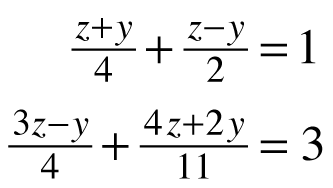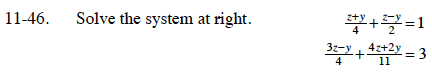Home > CCA2 > Chapter 11 > Lesson 11.2.1 > Problem11-46

11-46.

Solve the system below. Homework Help ✎Eliminate the denominators in both equations and simplify them before solving.

$4\left(\frac{z +y}{4}+\frac{z - y}{2} = 1\right)$

$44\left(\frac{3z-y}{4}+\frac{4z+2y}{11} = 3\right)$

(z + y) + 2(zy) = 4

11(3zy) + 4(4z + 2y) = 132

3zy = 4

54z − 3y = 132

Now finish solving this system of equations.